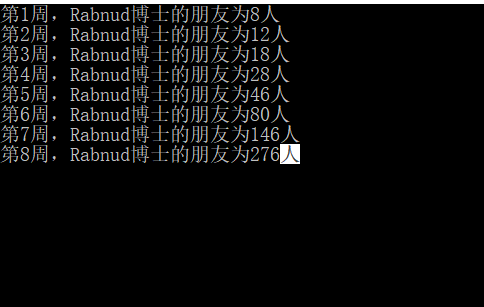• c语言三个数从小到大
千次阅读
2022-03-17 21:38:06

任意输入 3 个整数，编程实现对这 3 个整数由小到大进行排序

## 实现过程：

#include <stdio.h>
int main()
{
int a,b,c,t;    /*定义4个基本整型变量a、b、c、t*/
printf("Please input a,b,c:\n");    /*双引号内的普通字符原样输出并换行*/
scanf("%d,%d,%d",&a,&b,&c);    /*输入任意3个数*/
if(a>b)    /*如果a大于b,借助中间变量t实现a与b值的互换*/
{
t = a;
a = b;
b = t;
}
if(a>c)    /*如果a大于c,借助中间变景t实现a与c值的互换*/
{
t = a;
a = c;
c = t;
}
if(b>c)    /*如果b大于c,借助中间变量t实现b与c值的互换*/
{
t = b;
b = c;
c = t;
}
printf("The order of the number is:\n");
printf("%d,%d,%d",a,b,c);    /*输出函数顺序输出a、b、c的值*/
return 0;
}

输出结果

​
5,3,9
The order of the number is:
3,5,9

​

注意：

本实例使用 scanf("%d%d%d",&a,&b,&c); 从键盘中获得任意 3 个数。在输入数据时，在两个数据之间以一个或多个空格间隔，也可以用 Enter 健、Tab 键，不能用逗号作为两个数据间的分隔符。 如果用格式输入函数 scanf("％d,％d,%d",&a,&b,&c) 输入数据，两个数据之间要用“,”做间隔。排序算法
更多相关内容
• c语言三个数从小到大排序/输出 任意输入 3 个整数，编程实现对这 3 个整数由小到大进行排序。 （1)定义数据类型，本实例中 a、b、c、t 均为基本整型。 (2) 使用输入函数获得任意 3 个值赋给 a、b、c。 (3) 使用 if ...

## c语言三个数从小到大排序/输出

任意输入 3 个整数，编程实现对这 3 个整数由小到大进行排序。

（1)定义数据类型，本实例中 a、b、c、t 均为基本整型。
(2) 使用输入函数获得任意 3 个值赋给 a、b、c。
(3) 使用 if 语句进行条件判断，如果 a 大于 b，则借助于中间变量 t 互换 a 与 b 值， 依此类推比较 a 与 c、b 与 c，最终结果即为 a、b、c 的升序排列。
(4) 使用输出函数将 a、b、c 的值依次输出。

#include<stdio.h>
int main()
{
int a,b,c,t;
scanf("%d%d%d",&a,&b,&c);
if(a>b){
t=a;
a=b;
b=t;
}
if(a>c){
t=a;
a=c;
c=t;
}
if(b>c){
t=b;
b=c;
c=t;}
printf("%d %d %d",a,b,c);
return 0;
}

展开全文• c代码-C语言三个数从小到大排序/输出： 任意输入 3 个整数，编程实现对这 3 个整数由小到大进行排序。 冒泡排序的动画 https://haokan.baidu.com/v?vid=8080732080990475789&pd=bjh&fr=bjhauthor&type=video
• ## C语言三个数从小到大排列

万次阅读 多人点赞 2017-11-04 23:56:25
#include<stdio.h> int main() { int a,b,c,t; scanf("%d %d %d",&a,&b,&c); if(a>b) { t=b; ...#include<stdio.h>
int main()
{
int a,b,c,t;
scanf("%d %d %d",&a,&b,&c);
if(a>b)
{
t=b;
b=a;
a=t;
}
if(a>c)
{
t=c;
c=a;
a=t;
}
if(b>c)
{
t=c;
c=b;
b=t;
}
printf("%d->%d->%d\n",a,b,c);
return 0;
}  

展开全文• /*计算三个数的平均值的程序*/ #include<stdio.h> #include<math.h> int main() { int one,two,three;//声明三个变量 double four;//声明平均值变量 printf("整个程序可以用来计算三个数的正整数平均.../*计算三个数的平均值的程序*/
#include<stdio.h>
#include<math.h>
int main()
{
int one,two,three;//声明三个变量
double four;//声明平均值变量
printf("整个程序可以用来计算三个数的正整数平均值n"
"请输入三个数值，无所谓正数还是负数n"
"空格隔开，回车输入n");//提高程序可读性
scanf("%d %d %d",&one,&two,&three);//读取三个用户输入的数值
four=(abs(one)+abs(two)+abs(three))/3.0;//计算平均值
printf("平均值为%.16lf",four);//打印平均值在屏幕上

getchar();
getchar();
return 0;
}

版本1.1：增加程序可读性

风暴：C程序——计算三个数的平均值（增加程序可读性）​zhuanlan.zhihu.com附：标题图片来自360壁纸（网络图片作者未知）

展开全文c语言求三个数最大值
• 本文转自：...版权声明：本文为CSDN博主「Nicole20171103」的原创文章，遵循 CC 4.0 BY-SA 版权协议，转载请附上原文出处链接及本声明。 #include<stdio.h> int main() { int a,b,c,t; scanf("%d %...
• 三个数从小到大输出: 每两个比较一次，三个数的话就要比较三次。 代码如下; 刚开始学习c语言，继续加油。
• 题目要求：输入三个整数，按从小到大的顺序输出。 #include<stdio.h> int main() { int x,y,z,c; scanf("%d,%d,%d",&x,&y,&z); if (x>y) { c=x; x=y; y=c; } if(x>z) { c=x; x=z; z=c; } if...
• 主要给大家介绍了关于如何利用C语言实现3个数从小到大排序/输出的相关资料，文中通过示例代码介绍的非常详细，对大家学习或者使用C语言具有一定的参考学习价值，需要的朋友们下面来一起学习学习吧
• 从控制台输入三个整数a,b,c,实现对这 3 个整数由小到大进行排序 实现方法：使用 if 语句进行条件判断，如果 a 大于 b，则借助于中间变量 temp 互换 a 与 b 值， 依此类推比较 a 与 c、b 与 c，最终结果即为 a、b、c ...
• ## C语言：将三个数从小到大输出

千次阅读 多人点赞 2021-02-28 14:26:20
C语言：将三个数从小到大输出 #include <stdio.h> int main(void) { int a,b,c,t; printf(“请输入三个数：\n”); scanf("%d,%d,%d",&a,&b,&c); if(a>b) { t=a; a=b; b=t; } if(a>c) { t=a...
• printf("请输入三个整数：\n"); scanf("%d%d%d",&a,&b,&c); if(a>b){ // 3 2 1 t=a; // t=3 a=b; // a=2 b=t; // b=3 } if(a>c){ t=a; // t=2 a=c; // a=1 c=t; // c=2 } if(b&...
• 需求任意输入3整数，对这3整数由小到大进行排序，并将排序后的结果输出。源码// //@author:冲哥 //@date:2021/5/713:37 //@descript...cakephp
• 运用if语句进行三次判断 程序如下： #define _CRT_SECURE_NO_WARNINGS #include<... printf("请输入三个数（空格隔开）\n"); scanf("%d %d %d", &a, &b, &c); if (a > b) { ...
• 将3个数从小到大的顺序输出。 算数分析： 只需要将两个数两两比较，然后将小的写在前面，大的写在后面。 所以我们应该创建一转换变量，swap（int i，int j）将里面的数从小到大排序。 核心代码如下： ...
• 题目:输入三个整数.分别放在变量a,b,c中,然后把输入的数据重新按有小到大的顺序放在变量a,b,c中,最后输出a,b,c中的值! 代码: #include <stdio.h> int main() { int a , b , c , t; printf("input a , b , c:")...
• 任意输入 3 整数，编程实现对这 3 整数由小到大进行排序。实现过程：(1)定义数据类型，本实例中 a、b、c、t 均为基本整型。(2) 使用输入函数获得任意 3 值赋给 a、b、c。(3) 使用 if 语句进行条件判断，如果 a...
• #include int main() { int a, b, c; scanf("%d%d%d", &a, &b, &c); if (a > b) { int asd = a; a = b; b = asd; } if (a > c) { int asd = a;... } printf("从小到大为；%d %d %d", a, b, c); return 0; }
• 匿名网友:思路分析：先比较2个，取其中者与第三个数比较得出较者为最大，记为max.步骤如下：第一步：输入a,b,c.第二步：比较a,b.若a>b，则执行第三步；否则，执行第四步.第三步：比较a,c.若a>c，则...
• 三个数从小到大排序 #include <stidio.h> int main { int a,b,c,t; scanf(“%d%d%d”,&a,&b,&c); if(a>b) { a=t; t=b; b=a } if(a>c) { a=t; t=c; c=a; } if(b>c) { b=t; t=c; c=b; } ...
• //尽管使用，此代码不会是万能的，但你可以作为模板使用 #include<stdio.h> int main() { int a,b,c;... //输入你要排序的三个数 if(a>b) //假设第一个条件a>b { if(c>a) //.自然语言处理
• 案列主要是借助中间变量t来实现a与b值的互换。 #include <stdio.h> int main() { int a,b,c,t; /*定义4基本整型变量a、b、c、t*/ printf("Please input a,b,c:\n"); /*双引号内的普通字符原样输出并...if语句
• 输入三个数字，按从大到小输出，使用分支结构而没有使用循环或者数组，这是最初级的排序
• 资源共享，有什么错误和好的建议请指出 如果没资源分，我可以直接发给你）
• #include <stdio> int main() {float a,b,c,t; scanf("%f,%f,%f",&a,&b,&c); if(a>b) {t=a;a=b;b=t} if(b>c) {t=b;b=c;c=t} if(a>c) {t=a;a=c;c=t} printf("%f,%f,%f",a,b,c);...}...